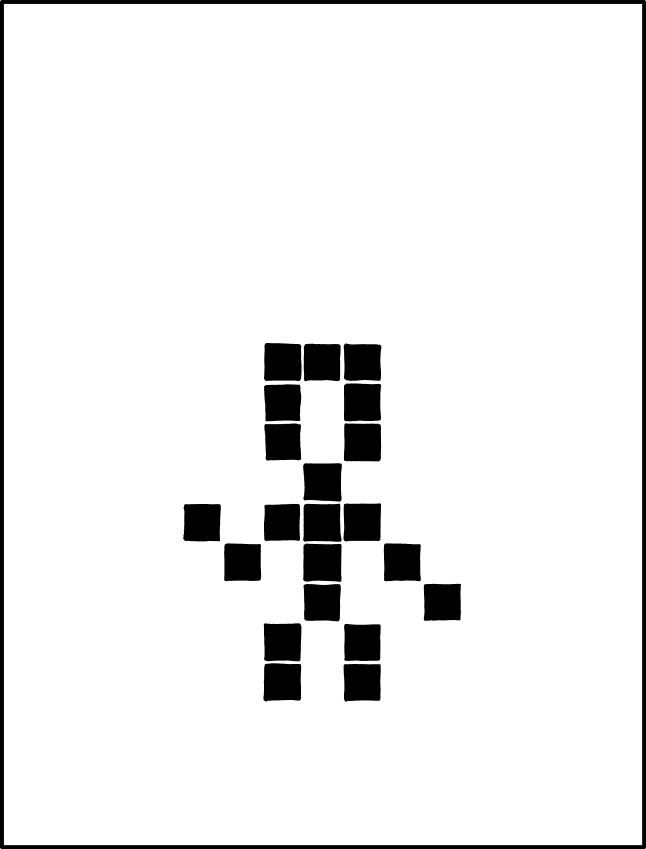#### Tech Support

Mon – Fri (10am – 5pm)

I’d been feeling demotivated all of yesterday. The week before, I’d been sick with an upset stomach, fever, and body ache. This week, the back pain had become unbearable. In isolation for the last four weeks due to COVID-19, I was unable to step out to get physiotherapy.
While going through all this, I came across xkcd’s RIP John Conway tribute comic.Yesterday evening, I sat down with my younger son to explore the Game of Life online. I was blown away by the simplicity and yet the beauty of the game. Some things are indeed timeless. I was also surprised that I had not come across it earlier. I blame that on a lack of formal Computer Science education 🙂
I thought that the best way to appreciate this game would be to quickly implement it in code. And I chose to do it not in my favourite languages, bash or C, but in Python. I’ve been using Python for the last fifteen years, since RedHat started using it in their system tools such as yum and system-config. However, I’ve never really programmed algorithms in Python. I’ve always written scripts or Flask web applications. I thought this would be a good way to teach myself the Pythonic way of implementing simple algorithms.
With a little help from Stack Overflow, it was simple enough to implement the game. Here is the help screen from the final implementation of the game:
```saurabh@saurabhj gol % python gol.py -h
usage: gol.py [-h] [--edge EDGE] [--debug] size interval pattern_file
The Game of Life
positional arguments:
size          The size of the matrix
interval      The interval between each iteration
pattern_file  The pattern file to be used
optional arguments:
-h, --help    show this help message and exit
--edge EDGE   The optional edge size. A negative edge is considered alive.
--debug       Debug the neighbourArray
```
Here are some sample runs of the game:
As I ran the game with various initial patterns, I realised that at the edge, my implementation was not behaving the same as BitStorm implementation. It was obvious that I hadn’t thought through the boundary conditions. I first superimposed the visible matrix onto a larger matrix. This was adequate for small starter patterns, but larger patterns would eventually bounce off the edge of the underlying matrix and reappear on the visible matrix. This bouncy edge had me really annoyed.
I then read online and realised that there are different boundary conditions possible in Life. I implemented two of these. One is a negative edge, and the other is an active edge. The cells in an active edge behave, when computing neighbours, like active cells. Such an edge is not as bouncy as a negative edge.
This was an evening well spent. My son lost interest when I started implementing the boundary conditions. He didn’t see what the big deal was. Perhaps, when I have time again, I will implement a toroidal boundary. Here is the source code as it currently stands.

```#!/usr/bin/python
import time
import subprocess
import argparse
def printArray(array, edge):
subprocess.call('clear',shell=True)
N = len(array)
edgeByte = u'\u2591'
if edge < 0:
edge = -edge
edgeByte = u'\u2593'
for i in range(N):
for j in range(N):
if i < edge or j < edge or i >= N - edge or j >= N - edge:
print edgeByte,
else:
if array[i][j] == 0:
print '.',
else:
print u'\u2588',
print
print
def neighbourCount(array, x, y, edge):
count = 0
N = len(array)
for i in [x - 1, x, x + 1]:
for j in [y - 1, y, y + 1]:
if i >= 0 and i < N and j >= 0 and j < N and not (i == x and y == j):
if edge < 0 and (i < abs(edge) or j < abs(edge) or i >= (N + edge) or j >= (N + edge)) and (array[x][y] == 1):
count += 1
else:
count += array[i][j]
return count
def makeNeighbourArray(array, neighbourArray, edge, debug):
N = len(array)
for i in range(N):
for j in range(N):
neighbourArray[i][j] = neighbourCount(array, i, j, edge)
if edge > 0 and (i < edge or j < edge or i >= N - abs(edge) or j >= N - abs(edge)):
neighbourArray[i][j] = 0
if debug:
print neighbourArray[i][j],
if debug:
print
# If the edge value is passed to this method, it assumes the edge is dead
def makeArrayFromNA(neighbourArray, array, edge):
stopIt = True
N = len(array)
for i in range(N):
for j in range(N):
if (neighbourArray[i][j] == 2 and array[i][j] == 1) or neighbourArray[i][j] == 3:
newval = 1
if edge != 0:
if i < edge or j < edge or i >= N - abs(edge) or j >= N - abs(edge):
if edge > 0:
newval = 0
else:
newval = 1
else:
newval = 0
if newval != array[i][j]:
stopIt = False
array[i][j] = newval
return stopIt
def insert_pattern(array, filename):
N = len(array)
file = open(filename, "r")
size = int(lines)
offset = int((N - size) / 2)
for i in range(1, size + 1):
for j in range(size):
array[offset + i][offset + j] = int(lines[i][j])
def main():
parser = argparse.ArgumentParser(description='The Game of Life')
parser.add_argument('size', help='The size of the matrix')
parser.add_argument('interval', help='The interval between each iteration')
parser.add_argument('pattern_file', help='The pattern file to be used')
parser.add_argument('--edge', help='The optional edge size. A negative edge is considered alive.', type=int, required=False, default=0)
args = parser.parse_args()
edge = int(args.edge)
if edge > 1:
edge = 1
if edge < 0:
edge = -1
N = int(args.size) + (abs(edge) * 2)
interval = float(args.interval)
iteration = 0
array = [[0 for i in range(N)] for j in range(N)]
neighbourArray = [[0 for i in range(N)] for j in range(N)]
insert_pattern(array, args.pattern_file)
stopIt = False
while not stopIt:
printArray(array, edge)
makeNeighbourArray(array, neighbourArray, edge, args.debug)
stopIt = makeArrayFromNA(neighbourArray, array, edge)
iteration += 1
print ("Iteration: " + str(iteration))
if interval:
time.sleep(interval)
else:
ignore = raw_input("Press Enter to continue:")
if __name__ == '__main__':
main()
```